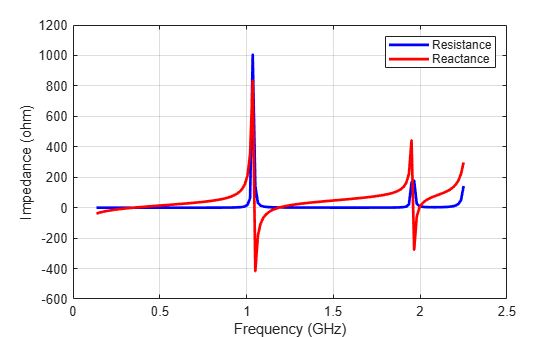# Double-Slot Cavity Patch on TMM10 Substrate

This example shows you how to create a custom slot cavity patch using custom antenna geometry and thick dielectric substrate. A double slot cavity patch consist of a double slot patch, backed by a cavity and probe-fed. The cavity is filled with TMM10 substrate. The cavity backing helps to reduce back radiation. You can use this antenna for microwave imaging by placing the antenna close to the human body.

Below is the picture of the fabricated slotted patch antenna.Fabricated slotted patch antenna(with permission from Antenna Lab, WPI)

### Create a Double Slot Patch

A double slot patch is not available as part of the Antenna Toolbox Library. However, you can create the geometry using basic rectangle shape primitive. You can put this information into a customAntennaGeometry antenna object and Boolean operation is performed to create the slots.

```rect1 = antenna.Rectangle('Length', 37e-3, 'Width', 37e-3); p1 = getShapeVertices(rect1); slot1 = antenna.Rectangle('Length', 2e-3, 'Width', 23e-3, ... 'Center', [-5e-3, 0],'NumPoints',[5 10 5 10]); p2 = getShapeVertices(slot1); slot2 = antenna.Rectangle('Length', 2e-3, 'Width', 23e-3, ... 'Center', [ 5e-3, 0],'NumPoints',[5 10 5 10]); p3 = getShapeVertices(slot2); feed1 = antenna.Rectangle('Length', 0.5e-3, 'Width', 0.5e-3, ... 'Center', [-17.25e-3 0]); p4 = getShapeVertices(feed1); ant = customAntennaGeometry; ant.Boundary = {p1,p2,p3,p4}; ant.Operation = 'P1-P2-P3+P4'; ant.FeedLocation = [-17.5e-3,0,0]; ant.FeedWidth = 0.5e-3; figure show(ant); ```### Provide Cavity Backing with Probe Feed

Use the slot patch created as an exciter for the cavity and enable probe feed. Below you see the patch antenna structure on an air substrate.

```c = cavity('Exciter', ant, 'Length', 57e-3, 'Width', 57e-3, 'Height', ... 6.35e-3, 'Spacing', 6.35e-3, 'EnableProbeFeed', 1); figure; show(c); ```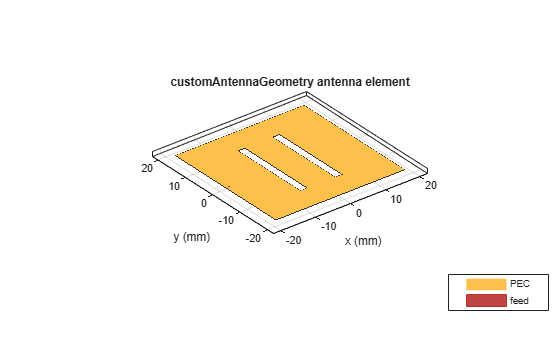### Calculate the Antenna Impedance

Calculate the antenna impedance over the range of 2.4 GHz to 3 GHz. From the figure, observe that the antenna resonates around 2.76 GHz.

```figure; impedance(c, linspace(2.4e9, 3.0e9, 61)); ```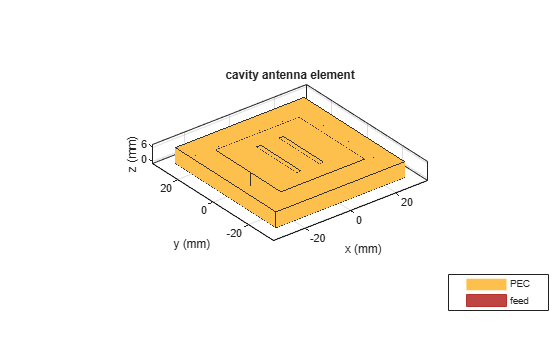### Visualize the Antenna Mesh

At the highest frequency of 2.2 GHz, the wavelength (lambda) in TMM10 dielectric is 43.6 mm. So the substrate thickness is lambda/7. So to make the thick substrate accurate thick substrate, two layers of tetrahedra are automatically generated.

```figure; mesh(c); ```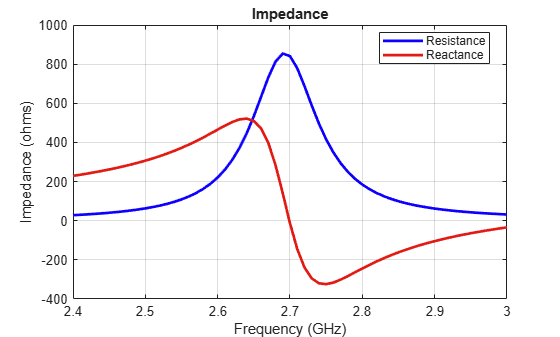Fill the space between the cavity and the patch with Rogers TMM10 substrate from the dielectric catalog.

```c.Substrate = dielectric('TMM10'); show(c); ```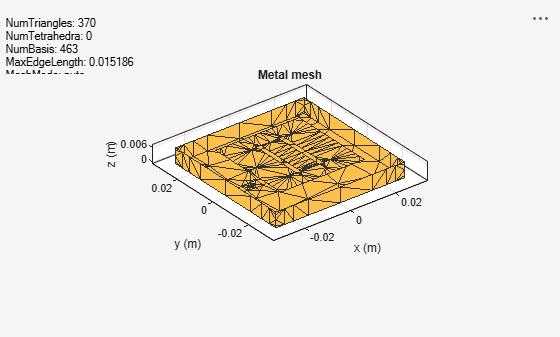### Meshing the Antenna

Mesh the antenna with a maximum edgelength of 3.5 mm.

```mesh(c,'MaxEdgelength',3.5e-3); ```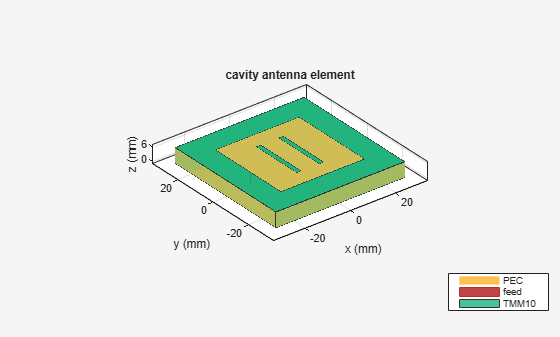### Calculate the Antenna Impedance

The effect of the dielectric constant is to move the resonance by a factor of sqrt(9.8) ~ 3, approximately. So antenna miniaturization is achieved by adding a dielectric substrate. However, as the dielectric constant of the substrate increases the antenna higher Q-factor creates a sharp resonance. Due to the large numbers of frequency steps involved, the results are pre-computed and stored. Only one of the highest frequency computations is shown.

```zl = impedance(c, 2.2e9); load cavitypatch; figure; plot(freq./1e9, real(Z), 'b', freq./1e9, imag(Z), 'r', 'LineWidth',2); xlabel('Frequency (GHz)'); ylabel('Impedance (ohm)'); legend('Resistance','Reactance'); grid on; ```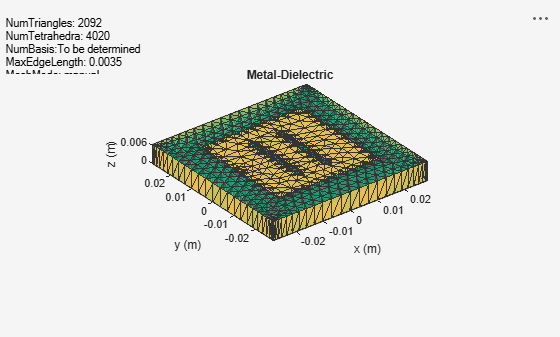### Fabricated Slot-patch Antenna

The double slot patch antenna was manufactured and its reflection coefficient was measured at the Antenna Lab in Worcester Polytechnic Institute (WPI). As seen from the plot below, a very good agreement is achieved at the lower frequency. At the upper frequency the difference in the reflection coefficient is around 3.5%. This could be due to the SMA connector present on the actual antenna or the frequency variation in the dielectric constant for the substrate.

```figure plot(freq./1e9,s11_meas,'-r','LineWidth',2); grid on; hold on plot(freq./1e9,s11_sim,'-b','LineWidth',2); grid on; xlabel('Freq (GHz)') ylabel('S11 (dB)') axis([0.5,2.2,-5,0]) title('Antenna S11 Data') legend('Measurement','Simulated','Location', 'best') ```